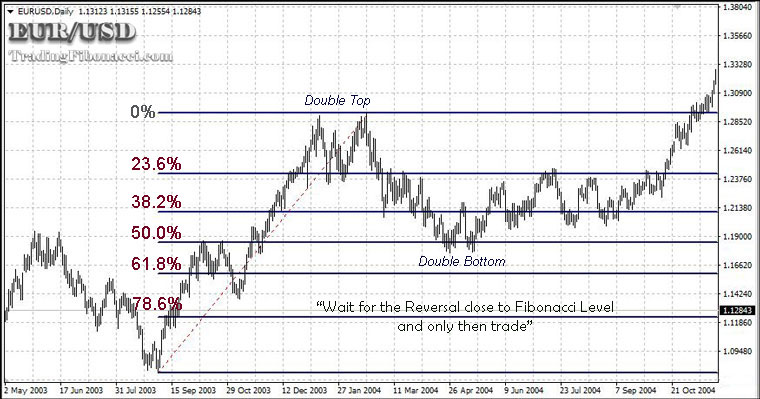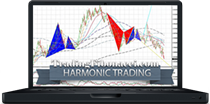The Fibonacci Retracement Tool

Fibonacci retracement is the most widely used technical analysis tool based on Fibonacci ratios. The Fibonacci retracement can be used when trading any financial market (Forex, Equities, Bonds or Commodities) in any timeframe. Preferably use the Fibonacci retracement only when trading liquid assets and apply it in timeframes longer than M30.

Short Explanation (Background) of the Fibonacci Retracement

As the price of a financial asset pulls back after an uptrend or a downtrend wave, that price pullback usually follows a certain mathematical relationship. The Fibonacci retracement calculates this relationship based on the Fibonacci ratios. The Fibonacci retracement uses the basic ratios (23.6%, 38.2%, 61.8%, and 78.6%) deriving from the Fibonacci's sequence of numbers, plus 50.0%.

The Key Fibonacci Ratios

Here is how the Fibonacci ratios are calculated:

• The Fibonacci ratio of 61.8% is considered a key level as it represents the 'golden ratio" or else the "golden mean". The 61.8% ratio can be found by dividing one Fibonacci number by the next Fibonacci number. For example: 144/233 = 0.618027.

• The Fibonacci ratio of 38.2% ratio is the second most important ratio and can be found by dividing one Fibonacci number by the Fibonacci number two places to the right. For example: 144/377 = 0.38196.

• The Fibonacci ratio of 23.6% ratio is the third most important ratio and can be found by dividing one Fibonacci number by the Fibonacci number three places to the right. For example: 144/610= 0.23606.

• The Fibonacci ratio of 78.6% is the result of subtracting 0.236 from 1.

How the Fibonacci Retracement Works

The Fibonacci retracement is applied by taking two extreme levels in a price chart. These levels are the peak and the bottom of a price move, that can be an uptrend or downtrend move

As it was mentioned before, the key Fibonacci ratios are 23.6%, 38.2%, and 61.8%. The Fibonacci retracement uses these three ratios plus the 50.0% and 78.6% ratios. That means 5 ratios in total: 23.6%, 38.2%, 50.0%, 61.8% and 78.6%. When the Fibonacci retracement tool is applied, these levels are visualized as horizontal lines between the peak and the bottom. These 5 horizontal lines represent possible support and resistance levels.

These ratios can be used for the identification of critical points that can cause reversals in the price of financial assets. It is important to mention that these Fibonacci retracement levels should be seen as Support & Resistance areas and not as hard support and resistance price levels.

• For an uptrend, put the 0.0 level of the retracement tool at the wave low and the 100.0 level at the wave high.

• For a downtrend, put the 100.0 level of the retracement tool at the high of the wave and the 0.0 level at the bottom.

If the price falls through one Fibonacci level it will likely proceed to the next Fibonacci level.

The following chart illustrates how traders can apply the Fibonacci retracement to identify possible support and resistance levels after an uptrend.

Chart: Using the Fibonacci retracement on EURUSDDistinguishing Strong and Moderate Trends

If you trade a strong trend, then you should expect pullbacks to the Fibonacci levels 23.6% and 38.2%. In normal trends, you should expect pullbacks to 50.0% or most commonly to 61.8% levels.

In general, give extra weight to 38.2% and 61.8% retracement levels.

Placing Stop-Loss orders

One of the key advantages when trading using the Fibonacci retracement is that you can identify the optimal stop-loss levels at a glance. After the price has reversed close to a Fibonacci level, place a stop-loss below the local low or above the local high.

Fibonacci Retracement tool in Trading Platforms

The Fibonacci retracement tool is available in all modern trading platforms and can be applied with a few clicks. To apply the Fibonacci Retracement tool in MetaTrader:

Basic Tips when Using The Fibonacci Retracement Tool

(1) Ignore the Fibonacci retracement during important news releases or after key fundamental changes.

(2) Prefer to apply the Fibonacci retracement on highly liquid financial assets (Forex majors, major indices, etc).

(3) Apply the Fibonacci retracement in timeframes above M30. Preferably use the H4 and D1 timeframes.

(4) The levels generated by the Fibonacci retracement should be seen as general Support & Resistance areas and not as hard support and resistance price levels.

(5) It is better to wait until a price reversal is already occurred close to a Fibonacci level, before opening a position.

(6) Give extra weight to 38.2% and 61.8% levels, these two Fibonacci levels are the most important retracement levels.

(7) You can apply multiple Fibonacci retracements on different waves and timeframes (one does not cancel the other).

(8) Finally, it is better to confirm any signal generated by the Fibonacci retracement with another tool. For example, you can apply the MACD in its standard settings. Use MACD above the M30 timeframe, preferably in H1 and H4 timeframes.

Conclusions Using the Fibonacci Retracement Tool

If you ask an experienced trader to tell you his opinion about the Fibonacci retracement, he will tell you either that it rocks or it is useless. Remember the truth is found always in the middle of two extreme opinions. The Fibonacci retracement is useful given that it will be applied selectively and carefully. Select to apply it on liquid assets, select to apply it on long timeframes and confirm its results with a reliable indicator. That is all you have to do!

Fibonacci retracement tool

### Online Forex BrokerseBOOKTRADING WORLD MARKETS USING PHI AND THE FIBONACCI NUMBERS (2018)

Complete Guide to Fibonacci Trading with Reference to Elliott Waves, Gann Numbers, and Harmonic Patterns### Forex Rebates

Start trading Forex and receive rebates for every trade you execute. Permanent participation, 100% free of any charge. Contact Forex Rebates today..### Chart PatternsIdentify and Trade Reliable Chart Patterns: